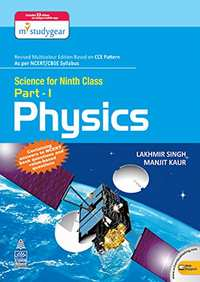Share

# Lakhmir Singh Physics Class 9 Solutions

## Solutions for Science for Class 9 Part 1 Physics

Shaalaa provides Lakhmir Singh Solutions for Class 9 Science and has all the answers for the questions given in Science for Class 9 Part 1 Physics. Shaalaa is surely a site that most of your classmates are using to perform in exams.

You can solve Science for Class 9 Part 1 Physics and use Shaalaa Lakhmir Singh Solutions for Class 9 Science to check your answers.

## Lakhmir Singh Solutions for Class 9 Science

Given below is chapter wise list for Lakhmir Singh Solutions for Class 9 Science. Select any chapter number to view solutions.

• Chapter 4: Work and energy
• Chapter 5: Sound

#### Lakhmir Singh Solutions for Class 9 Science## Chapters covered in Lakhmir Singh Solutions for Class 9 Science

#### Chapter 1: Motion

Shaalaa has a total of 161 questions are solved for this Chapter in Class 9 Science. Chapters covered in Motion are Derivation of Equations of Motion by Graphical Method, Describing Motion - Motion Along a Straight Line, Describing Motion - Uniform Motion and Nonuniform Motion, Distance and Displacement, Equations of Motion by Graphical Method - Equation for Position-time Relation, Equations of Motion by Graphical Method - Equation for Position-velocity Relation, Equations of Motion by Graphical Method - Equation for Velocity-time Relation, Graphical Representation of Motion - Distance-time Graphs, Graphical Representation of Motion - Velocity-time Graphs, Measuring the Rate of Motion - Speed with Direction, Motion (Introduction), Motion (Numerical), Rate of Change of Velocity, Uniform Circular Motion

#### Chapter 2: Force and Laws of Motion

Shaalaa has a total of 119 questions are solved for this Chapter in Class 9 Science. Chapters covered in Force and Laws of Motion are Balanced and Unbalanced Forces, Conservation of Momentum, First Law of Motion, Force and Laws of Motion (Numerical), Inertia and Mass, Mathematical Formulation of Second Law of Motion, Second Law of Motion, Third Law of Motion

#### Chapter 3: Gravitation

Shaalaa has a total of 179 questions are solved for this Chapter in Class 9 Science. Chapters covered in Gravitation are Archimedes' Principle, Concept of Free Fall, Free Fall - Motion of Objects Under the Influence of Gravitational Force of the Earth, Free Fall - to Calculate the Value of g, Gravitation, Importance of the Universal Law of Gravitation, Mass and Weight, Relative Density, Thrust and Pressure - Buoyancy, Thrust and Pressure - Pressure in Fluids, Thrust and Pressure - Why Objects Float Or Sink When Placed on the Surface of Water?, Universal Law of Gravitation

#### Chapter 4: Work and energy

Shaalaa has a total of 0 questions are solved for this Chapter in Class 9 Science. Chapters covered in Work and energy are Are Various Energy Forms Interconvertible?, Commercial Unit of Energy, Different Forms of Energy, Kinetic Energy, Law of Conservation of Energy, Potential Energy, Potential Energy of an Object at a Height, Rate of Doing Work, Scientific Conception of Work, Work and Energy (Introduction ), Work and Energy (Numericals), Work Done by a Constant Force, Work Done by a Energy, Work Done by a Power

#### Chapter 5: Sound

Shaalaa has a total of 0 questions are solved for this Chapter in Class 9 Science. Chapters covered in Sound are Applications of Ultrasound - SONAR, Production of Sound, Propagation of Sound, Range of Hearing in Humans, Reflection of Sound - Echo, Reflection of Sound - Reverberation, Sound Needs a Medium to Travel, Sound (Numerical), Sound Waves Are Longitudinal Waves, Speed of Sound in Different Media, Structure of the Human Ear (Auditory Aspect Only), Uses of Multiple Reflection of Sound

## Lakhmir Singh Solutions for Class 9 Science

Class 9 Lakhmir Singh solutions answers all the questions given in the Lakhmir Singh textbooks in a step-by-step process. Our Maths tutors have helped us put together this for our Class 9 Students. The solutions on Shaalaa will help you solve all the Lakhmir Singh Class 9 Science questions without any problems. Every chapter has been broken down systematically for the students, which gives fast learning and easy retention.

Shaalaa provides free Lakhmir Singh solutions for Class 9 Science. Shaalaa has carefully crafted Lakhmir Singh solutions for Class 9 Science that can help you understand the concepts and learn how to answer properly in your board exams. You can also share our link for free Class 9 Science Lakhmir Singh solutions with your classmates.

If you have any doubts while going through our Class 9 Science Lakhmir Singh solutions, then you can go through our Video Tutorials for Science. The tutorials should help you better understand the concepts.

S